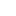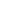# Disjoint Set Data Structure – Union Find Algorithm

What is Disjoint-set data structure?

• Represents a mathematics concept Set.
• A disjoint-set data structure, also called a union–find data structure or merge–find set.
• A disjoint-set data structure that keeps track of a set of elements partitioned into a number of disjoint or non-overlapping subsets.
• It provides near-constant-time operations to add new sets, to merge existing sets, and to determine whether elements are in the same set.
• Plays a key role in Kruskal’s algorithm for finding the minimum spanning tree of a graph.
• This can also be used to detect cycle in the graph.

How Disjoint Set is constructed:

• A disjoint-set forest consists of a number of elements each of which stores an id, a parent pointer
• The parent pointers of elements are arranged to form one or more trees, each representing a set.
• If an element’s parent pointer points to no other element, then the element is the root of a tree and is the representative member of its set.
• A set may consist of only a single element. However, if the element has a parent, the element is part of whatever set is identified by following the chain of parents upwards until a representative element (one without a parent) is reached at the root of the tree.Disjoint Set Operations:

MakeSet(X): This operation makes a new set by creating a new element with a parent pointer to itself. The parent pointer to itself indicates that the element is the representative member of its own set. The MakeSet operation has O(1) time complexity.

Find(X): follows the chain of parent pointers from x upwards through the tree until an element is reached whose parent is itself. This element is the root of the tree and is the representative member of the set to which x belongs, and may be x itself.

Union(x,y): Uses Find to determine the roots of the trees x and y belong to. If the roots are distinct, the trees are combined by attaching the root of one to the root of the other. If this is done naively, such as by always making x a child of y, the height of the trees can grow as O(n).

See the animation below for more understanding.disjoint-0
disjoint-1
disjoint-2
disjoint-3
disjoint-4
disjoint-5Code:

 import java.util.*; public class DisjointSet { static class Edge{ int source; int destination; public Edge(int source, int destination) { this.source = source; this.destination = destination; } } static class Graph{ int vertices; LinkedList[] adjList; ArrayList allEdges = new ArrayList<>(); Graph(int vertices){ this.vertices = vertices; adjList = new LinkedList[vertices]; for (int i = 0; i (); } } public void addEgde(int source, int destination){ Edge edge = new Edge(source, destination); adjList[source].addFirst(edge); allEdges.add(edge); //add to total edges } public void makeSet(int [] parent){ //Make set- creating a new element with a parent pointer to itself. for (int i = 0; i > map = new HashMap<>(); for (int i = 0; i list = map.get(parent[i]); list.add(i);//add vertex map.put(parent[i],list); }else{ ArrayList list = new ArrayList<>(); list.add(i); map.put(parent[i],list); } } //Print the Different sets Set set = map.keySet(); Iterator iterator = set.iterator(); while(iterator.hasNext()){ int key = iterator.next(); System.out.println("Set Id: " + key + " elements: " + map.get(key)); } } } public static void main(String[] args) { int vertices = 6; Graph graph = new Graph(vertices); graph.addEgde(0, 1); graph.addEgde(0, 2); graph.addEgde(1, 3); graph.addEgde(4, 5); graph.disjointSets(); } }

view raw
DisjointSet.java
hosted with ❤ by GitHub

Output

```Set Id: 0 elements: [0, 1, 2, 3]
Set Id: 4 elements: [4, 5]
```